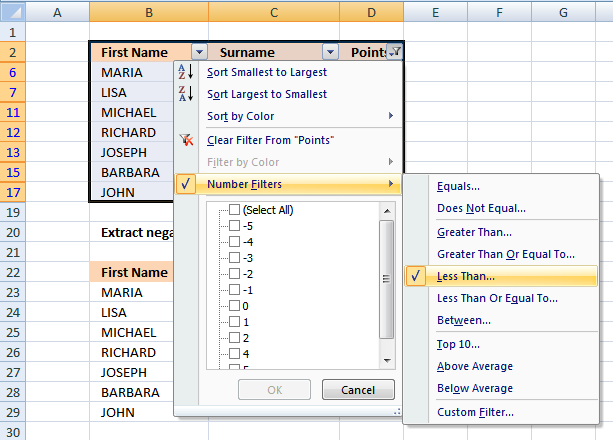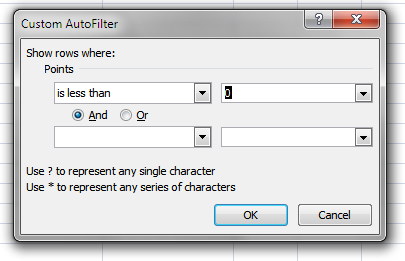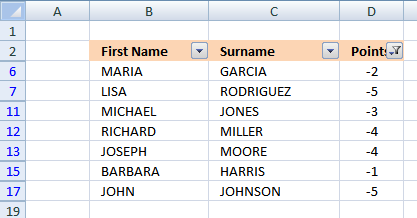Author: Oscar Cronquist Article last updated on February 20, 2019

Extract negative values and adjacent cells (array formula)
Extract negative values and adjacent cells (Excel Filter)Array formula in B23:

=INDEX(\$B\$3:\$D\$18, SMALL(IF(\$D\$3:\$D\$18<0, ROW(\$D\$3:\$D\$18)-MIN(ROW(\$D\$3:\$D\$18))+1, ""), ROW(A1)), COLUMN(A1)) + CTRL + SHIFT + ENTER

copied down as far as needed and then copied to the right as far as needed.

### Explaining array formula in cell B23

Step 1 - Filter negative values and return their corresponding row number

IF(\$D\$3:\$D\$18<0, ROW(\$D\$3:\$D\$18)-MIN(ROW(\$D\$3:\$D\$18))+1, "")

becomes

IF({1;5;0;-2;-5;2;4;4;-3;-4;-4;1;-1;0;-5;1}<0, ROW(\$D\$3:\$D\$18)-MIN(ROW(\$D\$3:\$D\$18))+1, "")

becomes

IF({FALSE; FALSE; FALSE; TRUE; TRUE; FALSE; FALSE; FALSE; TRUE; TRUE; TRUE; FALSE; TRUE; FALSE; TRUE; FALSE}, ROW(\$D\$3:\$D\$18)-MIN(ROW(\$D\$3:\$D\$18))+1, "")

becomes

IF({FALSE; FALSE; FALSE; TRUE; TRUE; FALSE; FALSE; FALSE; TRUE; TRUE; TRUE; FALSE; TRUE; FALSE; TRUE; FALSE}, {1; 2; 3; 4; 5; 6; 7; 8; 9; 10; 11; 12; 13; 14; 15; 16}, "")

and returns

{"";"";"";4;5;"";"";"";9;10;11;"";13;"";15;""}

Step 2 - Return the k-th smallest row number

SMALL(IF(\$D\$3:\$D\$18<0, ROW(\$D\$3:\$D\$18)-MIN(ROW(\$D\$3:\$D\$18))+1, ""), ROW(A1))

becomes

SMALL({"";"";"";4;5;"";"";"";9;10;11;"";13;"";15;""}, ROW(A1))

becomes

SMALL({"";"";"";4;5;"";"";"";9;10;11;"";13;"";15;""}, 1)

and returns 4.

Step 3 - Return value from cell range

INDEX(\$B\$3:\$D\$18, SMALL(IF(\$D\$3:\$D\$18<0, ROW(\$D\$3:\$D\$18)-MIN(ROW(\$D\$3:\$D\$18))+1, ""), ROW(A1)), COLUMN(A1))

becomes

INDEX(\$B\$3:\$D\$18, 4, 1)

becomes

INDEX({"MARGARET","ROBINSON",1; "DAVID","DAVIS",5; "MICHAEL","JONES",0; "MARIA","GARCIA",-2; "LISA","RODRIGUEZ",-5; "PATRICIA","JACKSON",2; "MARY","THOMAS",4; "THOMAS","TAYLOR",4; "MICHAEL","JONES",-3; "RICHARD","MILLER",-4; "JOSEPH","MOORE",-4; "LINDA","WHITE",1; "BARBARA","HARRIS",-1; "MARY","THOMAS",0; "JOHN","JOHNSON",-5; "DOROTHY","CLARK",1} 4, 1)

and returns "Maria" in cell B23.

extract negative values and adjacent cells.xls
(Excel 97-2003 Workbook *.xls)

### Extract negative values and adjacent cells (Excel Filter)

1. Select B2:D18
2. Click "Data" tab
3. Click "Filter" on the Ribbon
4. Click Black triangle in cell D25. Select "Number Filters"
6. Click "Less Than..."7. Type 0 (zero)
8. Click OK!MATCH(lookup_value;lookup_array, [match_type])
Returns the relative position of an item in an array that matches a specified value

IF(logical_test,[value_if:true],[value_if_false])
Checks whether a condition is met, and returns one value if TRUE, and another value if FALSE

SMALL(array,k) returns the k-th smallest row number in this data set.

INDEX(array,row_num,[column_num])
Returns a value or reference of the cell at the intersection of a particular row and column, in a given range

ROW(reference) returns the rownumber of a reference

COLUMN(reference)
returns the column number of a reference